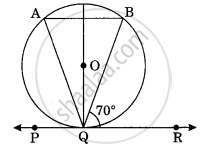# In figure, if PQR is the tangent to a circle at Q whose centre is O, AB is a chord parallel to PR and ∠BQR = 70°, then ∠AQB is equal to ______ - Mathematics

MCQ
Fill in the Blanks

In figure, if PQR is the tangent to a circle at Q whose centre is O, AB is a chord parallel to PR and ∠BQR = 70°, then ∠AQB is equal to ______• 20°

• 40°

• 35°

• 45°

#### Solution

In figure, if PQR is the tangent to a circle at Q whose centre is O, AB is a chord parallel to PR and ∠BQR = 70°, then ∠AQB is equal to 40°.

Explanation:

AB || PR

∴ ∠ABQ =∠BQR  ........[Alternate interior angles]

⇒ ∠ABQ = 70°

Also, ∠BQR = ∠BAQ  .......[Angles in alternate segment]

⇒ ∠BAQ = 70°

In ∆AQB,

∠BAQ + ∠ABQ + ∠AQB = 180°

⇒ 70° + 70° + ∠AQB = 180°

⇒ ∠AQB = 180° – 140° = 40°.

Concept: Tangent to a Circle
Is there an error in this question or solution?

#### APPEARS IN

NCERT Mathematics Exemplar Class 10
Chapter 9 Circles
Exercise 9.1 | Q 10 | Page 104
Share